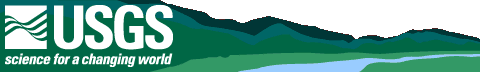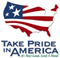# Techniques of Water-Resources Investigations Reports

Many USGS reports on water resources are now being served online. Most publications located at this site and other USGS sites can be located by subject, author, date, USGS series or publication series number by using the reports and thematic maps electronic Publications Warehouse. The Publications Warehouse will eventually be taking the place of this page.

## Book 1: Collection of Water Data by Direct Measurement

### Section D: Water quality

Chapter D1:  Water temperature—influential factors, field measurement, and data presentation, by H.H. Stevens, Jr., J.F. Ficke, and G.F. Smoot: USGS—TWRI Book 1, Chapter D1. 1975.

Chapter D2:  Guidelines for collection and field analysis of ground-water samples for selected unstable constituents, by W.W. Wood: USGS—TWRI Book 1, Chapter D2. 1976.

## Book 2: Collection of Environmental Data

### Section D: Surface geophysical methods

Chapter D1:  Application of surface geophysics to ground-water investigations, by A.A.R. Zhody, G.P. Eaton, and D.R. Mabey: USGS—TWRI Book 2, Chapter D1. 1974.

Chapter D2:   Application of seismic-refraction techniques to hydrologic studies, by F.P. Haeni: F.P. Haeni: USGS—TWRI Book 2, Chapter D2. 1988.

#### Section E: Subsurface geophysical methods

Chapter E1:  Application of borehole geophysics to water-resources investigations, by W.S. Keys and L.M. MacCary: USGS—TWRI Book 2, Chapter E1. 1971.

Chapter E2:   Borehole geophysics applied to ground-water investigations, by W.S. Keys: USGS—TWRI Book 2, Chapter E2. 1990.

Chapter F1:  Application of drilling, coring and sampling techniques to test holes and wells, by Eugene Shuter and W.E. Teasdale: USGS—TWRI Book 2, Chapter F1. 1989.

## Book 3: Applications of Hydraulics

### Section A: Surface-water techniques

Chapter A1:   General field and office procedures for indirect discharge measurements, by M.A. Benson and Tate Dalrymple: USGS—TWRI Book 3, Chapter A1. 1967.

Chapter A2:   Measurement of peak discharge by the slope-area method, by Tate Dalrymple and M.A. Benson: USGS—TWRI Book 3, Chapter A2. 1968.

Chapter A3:   Measurement of peak discharge at culverts by indirect methods, by G.L. Bodhaine: USGS—TWRI Book 3, Chapter A3. 1968.

Chapter A4:   Measurement of peak discharge at width contractions by indirect methods, by H.F. Matthai: USGS—TWRI Book 3, Chapter A4. 1967.

Chapter A5:   Measurement of peak discharge at dams by indirect methods, by Harry Hulsing: USGS—TWRI Book 3, Chapter A5. 1967.

Chapter A6:   General procedure for gaging streams, by R.W. Carter and Jacob Davidian: USGS—TWRI Book 3, Chapter A6. 1968.

Chapter A7:   Stage measurement at gaging stations, by T.J. Buchanan and W.P. Somers: USGS–TWRI Book 3, Chapter A7. 1968.

Chapter A8:   Discharge measurements at gaging stations, by T.J. Buchanan and W.P. Somers: USGS—TWRI Book 3, Chapter A8. 1969.

Chapter A9:   Measurement of time of travel in streams by dye tracing, by F.A. Kilpatrick and J.F. Wilson, Jr.: USGS—TWRI Book 3, Chapter A9. 1989.

Chapter A10:  Discharge ratings at gaging stations, by E.J. Kennedy: USGS—TWRI Book 3, Chapter A10. 1984.

Chapter A11:  Measurement of discharge by the moving-boat method, by G.F. Smoot and C.E. Novak: USGS—TWRI Book 3, Chapter A11. 1969.

Chapter A12:  Fluorometric procedures for dye tracing,revised, by J.F. Wilson, Jr., E.D. Cobb, and F.A. Kilpatrick: USGS—TWRI Book 3, Chapter A12. 1986.

Chapter A13:  Computation of continuous records of streamflow, by E.J. Kennedy: USGS—TWRI Book 3, Chapter A13. 1983.

Chapter A14:  Use of flumes in measuring discharge, by F.A. Kilpatrick and V.R. Schneider: USGS—TWRI Book 3, Chapter A14. 1983.

Chapter A15:  Computation of water-surface profiles in open channels, by Jacob Davidian: USGS—TWRI Book 3, Chapter A15. 1984.

Chapter A16:  Measurement of discharge using tracers, by F.A. Kilpatrick and E.D. Cobb: USGS—TWRI Book 3, Chapter A16. 1985.

Chapter A17:  Acoustic velocity meter systems, by Antonius Laenen: USGS—TWRI Book 3, Chapter A17. 1985.

Chapter A18:  Determination of stream reaeration coefficients by use of tracers, by F.A. Kilpatrick, R.E. Rathbun, Nobuhiro Yotsukura, G.W. Parker, and L.L. Delong: USGS—TWRI Book 3, Chapter A18. 1989.

Chapter A19:  Levels at streamflow gaging stations, by E.J. Kennedy: USGS—TWRI Book 3, Chapter A19. 1990.

Chapter A20:  Simulation of soluble waste transport and buildup in surface waters using tracers, by F.A. Kilpatrick: USGS—TWRI Book 3, Chapter A20. 1993.

Chapter A21:  Stream-gaging cableways, by C.R. Wagner: USGS—TWRI Book 3, Chapter A21. 1995.

### Section B: Ground-water techniques

Chapter B1:  Aquifer-test design, observation, and data analysis, by R.W. Stallman: USGS—TWRI Book 3, Chapter B1. 1971.

Chapter B2:  Introduction to ground-water hydraulics, by G.D. Bennett: USGS—TWRI Book 3, Chapter B2. 1976.

Chapter B3:  Type curves for selected problems of flow to wells in confined aquifers, by J.E. Reed: USGS—TWRI Book 3, Chapter B3. 1980.

Chapter B4:   Regression modeling of ground-water flow, by R.L. Cooley and R.L. Naff: USGS—TWRI Book 3, Chapter B4. 1990.

Chapter B5:  Definition of boundary and initial conditions in the analysis of saturated ground-water flow systems—an introduction, by 0. Lehn Franke, T.E. Reilly, and G.D. Bennett: USGS—TWRI Book 3, Chapter B5. 1987.

Chapter B6:  The principle of superposition and its application in ground-water hydraulics, by T.E. Reilly, O. Lehn Franke, and G.D. Bennett: USGS—TWRI Book 3, Chapter B6. 1987.

Chapter B7:  Analytical solutions for one-, two-, and three-dimensional solute transport in ground-water systems with uniform flow, by E.J. Wexler: USGS—TWRI Book 3, Chapter B7. 1992.

Chapter B8:   System and boundary conceptualization in ground-water flow simulation, by T.E. Reilly: USGS—TWRI Book 3, Chapter B8. 2001.

### Section C: Sedimentation & erosion techniques

Chapter C1:   Fluvial sediment concepts, by H.P. Guy: USGS—TWRI Book 3, Chapter C1. 1970.

Chapter C2:   Field methods for measurement of fluvial sediment, by T.K. Edwards and G.D. Glysson: USGS—TWRI Book 3, Chapter C2. 1999.

Chapter C3:   Computation of fluvial-sediment discharge, by George Porterfield: USGS—TWRI Book 3, Chapter C3. 1972.

## Book4: Hydrologic Analysis and Interpretation

### Section A: Statistical analysis

Chapter A1:  Some statistical tools in hydrology, by H.C. Riggs: USGS—TWRI Book 4, Chapter A1. 1968.

Chapter A2:  Frequency Curves, by H.C. Riggs: USGS—TWRI Book 4, Chapter A2. 1968.

Chapter A3:  Statistical methods in water resources, by D.R. Helsel and R.M. Hirsch: USGS—TWRI Book 4, Chapter A3. 2002.—ONLINE ONLY

### Section B: Surface water

Chapter B1:  Low-flow investigations, by H.C. Riggs: USGS—TWRI Book 4, Chapter B1. 1972.

Chapter B2:  Storage analyses for water supply, by H.C. Riggs and C.H. Hardison: USGS—TWRI Book 4, Chapter B2. 1973.

Chapter B3  Regional analyses of streamflow characteristics, by H.C. Riggs: USGS—TWRI Book 4, Chapter B3. 1973.

#### Section D: Interrelated phases of the hydrologic cycle

Chapter D1:  Computation of rate and volume of stream depletion by wells, by C.T. Jenkins: USGS—TWRI Book 4, Chapter D1. 1968.

## Book 5: Laboratory Analysis

### Section A: Water analysis

Chapter A1:  Methods for determination of inorganic substances in water and fluvial sediments, by M.J. Fishman and L.C. Friedman, Editors: USGS—TWRI Book 5, Chapter A1. 1989.

Chapter A2:  Determination of minor elements in water by emission spectroscopy, by P.R. Barnett and E.C. Mallory, Jr.: USGS—TWRI Book 5, Chapter A2. 1971.

Chapter A3:  Methods for the determination of organic substances in water and fluvial sediments, by R.L. Wershaw, M.J. Fishman, R.R. Grabbe and L.E. Lowe: USGS—TWRI Book 5, Chapter A3. 1987.

Chapter A4:  Methods for collection and analysis of aquatic biological and microbiological samples, by L.J. Britton and P.E. Greeson, Editors: USGS—TWRI Book 5, Chapter A4. 1989.

Chapter A5:  Methods for determination of radioactive substances in water and fluvial sediments, by L.L. Thatcher, V.J. Janzer, and K.W. Edwards: USGS—TWRI Book 5, Chapter A5. 1977.

Chapter A6:  Quality assurance practices For the chemical and biological analyses of water and fluvial sediments, by L.C. Friedman and D.E. Erdmann: USGS—TWRI Book 5, Chapter A6. 1982.

### Section C: Sediment analysis

Chapter C1:  Laboratory theory and methods for sediment analysis, by H.P. Guy: USGS—TWRI Book 5, Chapter C1. 1969.

## Book 6: Modeling Techniques

### Section A: Ground water

Chapter A1:  A modular three-dimensional finite-difference ground-water flow model, M.G. McDonald and A.W. Harbaugh: USGS—TWRI Book 6, Chapter A1. 1988.

Chapter A2:  Documentation of a computer program to simulate aquifer-system compaction using the modular finite-difference ground-water flow model, by S.A. Leake and D.E. Prudic: USGS—TWRI Book 6, Chapter A2. 1991.

Chapter A3:  A modular finite-element model (MODFE) for areal and axisymmetric ground-water-flow problems, Part 1: Model description and user's manual, by L.J. Tork: USGS—TWRI Book 6, Chapter A3. 1993.

Chapter A4:  A modular finite-element model (MODFE) for areal and axisymmetric ground-water-flow problems, Part 2: Derivation of finite-element equations and comparisons with analytical solutions, by R.L. Cooley: USGS—TWRI Book 6, Chapter A4. 1992.

Chapter A5:  A modular finite-element model (MODFE) for areal and axisymmetric ground-water-flow problems, Part 3: Design philosophy and programming details, by L.J. Torak: USGS—TWRI Book 6, Chapter A5, 1993.

Chapter A6:  A coupled surface-water and ground-water flow model (MODBRANCH) for simulation of stream-aquifer interaction, by E.D. Swain and E.J. Wexler: USGS—TWRI Book 6, Chapter A6, 1996.

Chapter A7:  User's Guide to SEAWAT: A computer program for simulation of three-dimensional variable-density ground-water flow, by Weixing Guo and Christian D. Langevin: USGS—TWRI Book 6, Chapter A7, 2002.

## Book 7: Automated Data Processing and Computations

### Section C: Computer programs

Chapter C1:   Finite-difference model for aquifer simulation in two dimensions with results of numerical experiments, by P.C. Trescott, G.F. Pinder and S.P. Larson: USGS—TWRI Book 7, Chapter C1. 1976.

Chapter C2:   Computer model of two-dimensional solute transport and dispersion in ground water, by L.F. Konikow and J.D. Bredehoeft: USGS—TWRI Book 7, Chapter C2. 1978.

Chapter C3:  A model for simulation of flow in singular and interconnected channels, by R.W. Schaffranek, R.A. Baltzer and D.E. Goldberg: USGS—TWRI Book 7, Chapter C3. 1981.

## Book 8: Instrumentation

### Section A: Instruments for measurement of water level

Chapter A1:   Methods of measuring water levels in deep wells, by M.S. Garber and F.C. Koopman: USGS—TWRI Book 8, Chapter A1. 1968.

Chapter A2:   Installation and service Manual for U.S. Geological Survey manometers, by J.D. Craig: USGS—TWRI Book 8, Chapter A2. 1983.

Chapter A3:   Use of submersible pressure transducers in water-resources investigations, by L.A. Freeman, M.C. Carpenter, D.O. Rosenberry, J.P. Rousseau, Randy Unger, and John S. McLean: USGS—TWRIBook 8, Chapter A3, 2004

### Section B: Instruments for measurement of discharge

Chapter B2:   Calibration and maintenance of vertical-axis type current meters, by G.F. Smoot and C.E. Novak: USGS—TWRI Book 8, Chapter B2. 1968.

## Book 9: Handbooks for Water-Resources Investigations

Chapter A1:  Preparations for water sampling, by F.D. Wilde, D.B. Radtke, Jacob Gibs, and R.T. Iwatsubo: USGS—TWRI Book 9, Chapter A1. 1998.

Chapter A2:  Selection of equipment for water sampling, edited by F.D. Wilde, D.B. Radtke, Jacob Gibs, and R.T. Iwatsubo, Editors: USGS—TWRI Book 9, Chapter A2. 1998.

Chapter A3:  Cleaning of equipment for water sampling, edited by F.D. Wilde, D.B. Radtke, Jacob Gibs, and R.T. Iwatsubo: USGS—TWRI Book 9, Chapter A3. 1998.

Chapter A4:  Collection of water samples, edited by F.D. Wilde, D.B. Radtke, Jacob Gibs, and R.T. Iwatsubo: USGS—TWRI Book 9, Chapter A4. 1999.

Chapter A5:  Processing of water samples, edited by F.D. Wilde, D.B. Radtke, Jacob Gibs, and R.T. Iwatsubo: USGS—TWRI Book 9, Chapter A5. 1999.

Chapter A6:  Field measurements, edited by F.D. Wilde and D.B. Radtke (Section 6.6--2nd edition--9/2001): USGS—TWRI Book 9, Chapter A6. 1998.

Chapter A7:  Biological indicators, edited by D.N. Myers and F.D. Wilde: USGS—TWRI Book 9, Chapter A7. 1997.

Chapter A8:  Bottom-material samples, by D.B. Radtke: USGS—TWRI Book 9, Chapter A8. 1997.

Chapter A9:  Safety in field activities, by S.L. Land and R.G. Fay:USGS—TWRI Book 9, Chapter A9. 1997.

 AccessibilityFOIAPrivacyPolicies and Notices U.S. Department of the Interior, U.S. Geological Survey Persistent URL: http://pubsdata.usgs.gov/pubs/twri/index090905.html Page Contact Information: USGS Publishing Network Last modified: Monday, March 23 2020, 03:58:12 PM